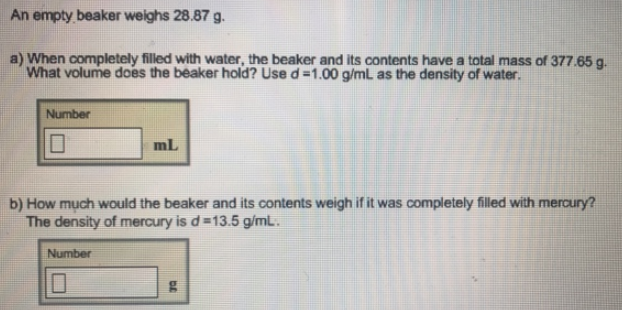# Problem: An empty beaker weighs 28.87 g. a) When completely filled with water, the beaker and its contents have a total mass of 37765 g. What volume does the beaker hold? Use d = 1.00 g/mL as the density of water.b) How much would the beaker and its contents weigh if it was completely filled with mercury? The density of mercury is d = 13.5 g/mL.

###### FREE Expert Solution###### Problem Details

An empty beaker weighs 28.87 g.

a) When completely filled with water, the beaker and its contents have a total mass of 37765 g. What volume does the beaker hold? Use d = 1.00 g/mL as the density of water.

b) How much would the beaker and its contents weigh if it was completely filled with mercury? The density of mercury is d = 13.5 g/mL.